• ＃＃＃ 用法除了常规 sub2ind 中提供的功能外，还提供了以下两个用例： ... A = randint(3, 4, 2) sub2ind(size(A), [2 1 2]') == sub2ind(size(A), 2, 1, 2) ## 示例 2 sub2ind(size(A), [2 4]')matlab
• 此函数的操作方式与“sub2ind”方法相同。 但是，这种方法要快得多。 它与 sub2ind.m 代码非常相似。 例子 ： >> a = [ 50 50 50 ]; >> b = [ 1 30 49 ]; >> x = sub2ind(a,b,b,b) x = 1 73980 122449 >> xx = sub...matlab
• MATLAB中ind2subsub2ind这对函数，是互逆的一对函数。ind2sub把数组或者矩阵的线性索引转化为相应的下标；sub2ind则正好相反，将下标转化为线性索引。 ind2sub的函数参考： [ij] = ind2sub(siz,IND) [I1,I2,I3,...
MATLAB中ind2sub和sub2ind这对函数，是互逆的一对函数。ind2sub把数组或者矩阵的线性索引转化为相应的下标；sub2ind则正好相反，将下标转化为线性索引。 ind2sub的函数参考：
[ij] = ind2sub(siz,IND)
[I1,I2,I3,...,In] = ind2sub(siz,IND)

sub2ind的函数参考：
linearInd =sub2ind(matrixSize, rowSub, colSub)
linearInd = sub2ind(arraySize, dim1Sub,dim2Sub, dim3Sub, ...)

上述的函数参考中，第一个都是二维的情况，第二个扩展到了多维空间。
matlab数组中元素引用有三种方式： 1.索引：就是由一个整数表示数组中的某一数据，Matlab中按照列进行排序 2.下标：如果是二维，就是行号和列号 3.布尔数
sub2ind函数：就是将下标转换为索引 ind2sub函数：就是在指定矩阵尺寸(size)前提下将给定的索引（index）转化成行列形式 例：
A = magic(2)
1  3
4  2

因为matlab中矩阵按照列进行排列，所以对应元素的索引为：
     1  3
2  4

下面使用sub2ind函数查看对应的索引：
sub2ind(size(A),2,1)
2

搜索矩阵A的第二行第一列对应的索引号2。
[i,j] = ind2sub(size(A),3)
i=1,j=2

上式即求矩阵A中第三个元素对应的行、列索引号（矩阵中的位置），矩阵A中第三个元素是3，位于第一行第二列。因为A是一个二维矩阵，所以用两个参数来接收返回值。
A(sub2ind(size(A),1,2))
3

参考链接： http://blog.sina.com.cn/s/blog_7054a1960102vyby.html
展开全文matlab
• sub2ind函数 官方链接） 2sub2ind函数的语法 有以下两种形式 （1）ind = sub2ind(sz, row, col) （2ind = sub2ind(sz, I1, I2,…,In) 本文主要对第一种形式进行python实现。 3、sub2ind函数的参数说明 输入...
（一）Matlab中sub2ind函数
1、sub2ind函数的功能
将下标转换为线性索引。
2、sub2ind函数的语法
有以下两种形式 （1）ind = sub2ind(sz, row, col) （2）ind = sub2ind(sz, I1, I2,…,In)
本文主要对第一种形式进行python实现。
3、sub2ind函数的参数说明
输入参数：
sz - 数组的大小（正整数向量）
数组的大小，指定为正整数向量。此向量的每个元素指示对应维度的大小。例如，[2 3 4] 定义 2×3×4 数组。
数据类型： single | double | int8 | int16 | int32 | int64 | uint8 | uint16 | uint32 | uint64
row - 行下标 （标量|向量|矩阵|多维数组）
行下标，指定为标量、向量、矩阵或多维数组。row 的大小必须与 col 的大小相同。
数据类型： single | double | int8 | int16 | int32 | int64 | uint8 | uint16 | uint32 | uint64
col - 列下标 （标量|向量|矩阵|多维数组）
列下标，指定为标量、向量、矩阵或多维数组。col 的大小必须与 row 的大小相同。
数据类型： single | double | int8 | int16 | int32 | int64 | uint8 | uint16 | uint32 | uint64
I1, I2,…,In - 多维下标（标量|向量|矩阵|多维数组）
多维下标，指定为标量、向量、矩阵或多维数组。每个数组 I1,I2,…,In 必须具有相同的大小。
数据类型： single | double | int8 | int16 | int32 | int64 | uint8 | uint16 | uint32 | uint64
输出参数：
ind - 线性索引（标量 |向量|矩阵|多维数组）
线性索引，指定为标量、向量、矩阵或多维数组。ind 的大小与输入 row、col 或 I1,I2,…,In 的大小相同。
数据类型： double
4、sub2ind函数说明
（1）ind = sub2ind(sz, row, col)：针对大小为sz的矩阵返回由row和col指定的行列下标的对应线性索引ind。此处，sz是包含两个元素的向量，其中sz(1)指定行数，sz(2)指定列数。
（2）ind = sub2ind(sz, I1, I2,…,In)：针对大小为sz的多维数组返回由n个数组I1, I2,…,In指定的多维下标的对应线性索引ind。此处，sz是包含n个元素的向量，用于指定每个数组维度的大小。
5、sub2ind函数示例
3x3的矩阵从下标到线性索引的映射如下所示。（1）在3*3矩阵中指定行下标和列下标，将下标转换为线性索引。
>> row = [1 2 3 1];
>> col = [2 2 2 3];
>> sz = [3 3];
>> ind = sub2ind(sz, row, col)

ind =

4     5     6     7

（2）将下标转换为三维数组的线性索引 2x2x2的数组从下标到线性索引的映射如下所示。在2x2x2数组中指定行下标，列下标和页下标，将下标转换为线性索引。
>> I1 = [1 2 1 2];
>> I2 = [2 2 1 1];
>> I3 = [1 1 2 2];
>> sz = [2 2 2];
>> ind = sub2ind(sz, I1, I2, I3)

ind =

3     4     5     6

（二）使用Python实现sub2ind函数
在将Matlab的sub2ind函数改写成Python的过程中遇到了很多问题，查看相关资料，可以从Python中的ravel_multi_index函数的功能着手进行深入了解。
1、重点说明
（i）Python中下标是以 0 作为开始的；
（ii）在numpy中，访问的以行为主的元素，而不是以列为主元素；
也就是说， 在Matlab中的索引矩阵为：
                      1  4  7

Matlab_ind = 2 5 8 以列为主进行索引排序的。 3 6 9
				      0   1  2

Python_ind = 3 4 5 以行为主进行索引排序的。 6 7 8
2、详细代码展示
def col_sub2ind(array_shape, rows, cols):
ind = rows + cols*array_shape
if ind < 0:
ind = -1
print("Index Value ERROR!")
elif ind >= array_shape*array_shape:
ind = -1
print("Index Value OVERFLOW!")
else:
return ind

3、参数说明
array_shape：可使用array.shape； rows：数组的行数； cols：数组的列数；
4、示例
import numpy as np
# define a numpy array A
A = np.array([[1, 2, 3], [2, 9, 8], [5, 6, 7]])
print(A)

[[1 2 3]
[2 9 8]
[5 6 7]]

ind = col_sub2ind(A.shape, 2, 1)
print("ind = ", ind)

ind = 5

【注意】：ind的值为对应位置的索引值，不是数组中的元素值。
剖析：
数组A ————————>数组A对应的索引ind 1 2 3 ——————————> 0 3 6 2 9 8 ——————————> 1 4 7 5 6 7 ——————————> 2 5 8
因此当调用函数col_sub2ind(A.shape, 2, 1)，即想要获取数组A第2行，第1列元素A[2, 1] = 6的索引值时，ind = 5。
至此，我们就得到了numpy数组一列为主进行排序的对应位置的索引值。
PS：但是，该方法只是实现了给出一个对应行、列元素的索引值，Matlab中该函数可以同时得到多个元素位置的索引值，因此，col_sub2ind 函数还需要继续优化，只是，能够满足目前项目的要求。
展开全文python numpy matlab
• sub2ind官方解释 sub2ind(arraySize,dim1Sub,dim2Sub,dim3Sub, ...)为大小是arraySize的 N 维数组的每个维度返回与指定的下标等效的线性索引。arraySize输入是包含 n 个元素的矢量，指定数组中的维数。dimNSub输入...
sub2ind官方解释
sub2ind(arraySize, dim1Sub, dim2Sub, dim3Sub, ...) 为大小是 arraySize 的 N 维数组的每个维度返回与指定的下标等效的线性索引。arraySize 输入是包含 n 个元素的矢量，指定数组中的维数。dimNSub 输入是正整数标量或矢量，指定矩阵的一个或多个行-列下标。
我的理解 arraySize 则是一个数组，dim1Sub 是一个位置即可以是行坐标值，也可以二维坐标。dim2Sub 是一个位置即可以是列坐标值，也可以二维坐标。
荔枝：
A=[1:3;4:6] D=sub2ind(size(A),2,3)
结果：
A =
1     2     3      4     5     6
D =
6
分析：则结果是来是来自与第二行第三列的位置值， 注意：matlab中矩阵的存储是按列存储的顺序。

荔枝：
A=[1:3;4:6] D=sub2ind(size(A),[1,3],[2,3])
结果：
错误使用 sub2ind (line 43) 下标超出范围。
看了这篇大牛写的文章，明白了自己出错。

D=sub2ind(size(A),[1,3],[2,3]) 是索引出[1,2]和[3,3]的坐标
荔枝：
A=[1:3;4:6;7:9] D=sub2ind(size(A),[1,2],[3,3])
结果：
A =
1     2     3      4     5     6      7     8     9
D =
7     8
分析 sub2ind返回值是坐标的位置值


展开全文• squeeze, ind2sub, sub2ind 觉得有用的话,欢迎一起讨论相互学习~Follow Me squeeze 删除单维度–B=squeeze(A) B = squeeze(A)返回一个与A元素相同，但去掉了所有单维度的数组B。单例维度是指size(A,dim) = 1的任何...
一起来学matlab-matlab学习笔记11
11_2 高维数组处理和运算
squeeze, ind2sub, sub2ind
觉得有用的话,欢迎一起讨论相互学习~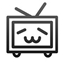squeeze
删除单维度–B=squeeze(A) B = squeeze(A)返回一个与A元素相同，但去掉了所有单维度的数组B。单例维度是指size(A,dim) = 1的任何维度。二维阵列不受squeeze函数的影响;如果A是一个行或列向量或标量(1×1)的值，那么B = A。
从数组中去掉单维度
创建一个(213)的数组，然后去掉单维度，使其成为一个(2*3)的矩阵

>> y = rand(2,1,3)
z = squeeze(y)

y(:,:,1) =

0.8147
0.9058

y(:,:,2) =

0.1270
0.9134

y(:,:,3) =

0.6324
0.0975

z =

0.8147    0.1270    0.6324
0.9058    0.9134    0.0975

sub2ind
将下标转换为线性索引
语法
linearInd = sub2ind(matrixSize, rowSub, colSub)linearInd = sub2ind(arraySize, dim1Sub, dim2Sub, dim3Sub, …)
说明
linearInd = sub2ind(matrixSize, rowSub, colSub) 为大小是 matrixSize 的矩阵返回与行和列下标 rowSub 和 colSub 等效的线性索引。matrixSize 输入是一个包含 2 个元素的向量，该向量以 * [nRows, nCols] 形式指定矩阵中的行和列数。rowSub 和 colSub 输入是正整数标量或向量，指定矩阵的一个或多个行-列下标对组。  linearInd = sub2ind(arraySize, dim1Sub, dim2Sub, dim3Sub, …) 为大小是 arraySize 的 N 维数组的每个维度返回与指定的下标等效的线性索引。arraySize 输入是包含 n 个元素的向量，指定数组中的维数。dimNSub 输入是正整数标量或向量，指定矩阵的一个或多个行-列下标。  所有下标输入可以是 single、double 或任意整数类型。linearInd 输出始终都为类 double。  如果需要，sub2ind 假定未指定的后续下标是 1。
三维数组的线性索引
将三维数组的索引转换为单个线性索引。

创建数组 A，并确定对应于 (2,1,2) 位置的元素的线性索引。

A = rand(3,4,2);
linearInd = sub2ind(size(A),2,1,2)
linearInd = 14
检查这两个索引版本是否引用 A 的同一元素。

A(2,1,2)
ans = 0.4854
A(14)
ans = 0.4854

ind2sub
线性索引的下标
语法
[I,J] = ind2sub(siz,IND) [I1,I2,I3,…,In] = ind2sub(siz,IND)
说明
ind2sub 函数确定与数组的单个索引对应的等效下标值。
[I,J] = ind2sub(siz,IND) 返回矩阵 I 和 J，其中包含与矩阵 IND（大小为 siz 的矩阵）中的每个线性索引对应的对等行下标和列下标。siz 是一个包含 ndim(A) 个元素（此示例为 2 个元素）的向量，其中 siz(1) 为行数，siz(2) 为列数。
注意 对于矩阵，[I,J] = ind2sub(size(A),find(A>5)) 与 [I,J] = find(A>5) 返回相同的值。
[I1,I2,I3,…,In] = ind2sub(siz,IND) 返回 n 个下标数组 I1,I2,…,In，其中包含等效于 IND（大小为 siz 的数组）的多维数组下标。siz 是一个指定每个数组维度大小的 n 元素向量。
IND 输入可以是 single、double 或任意整数类型。输出始终属于 double 类。
二维矩阵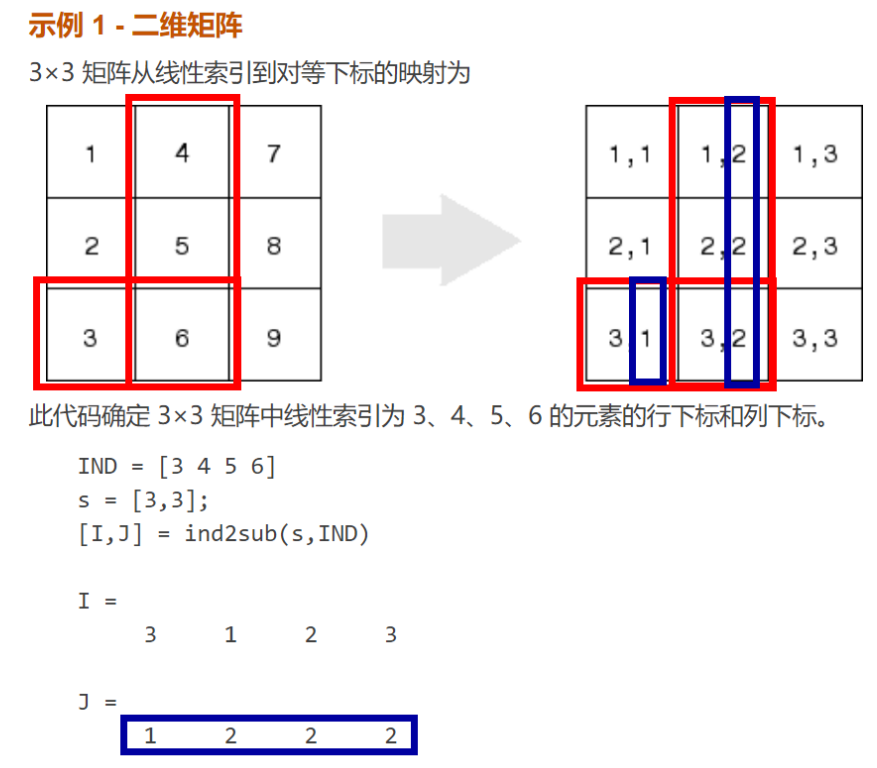三维矩阵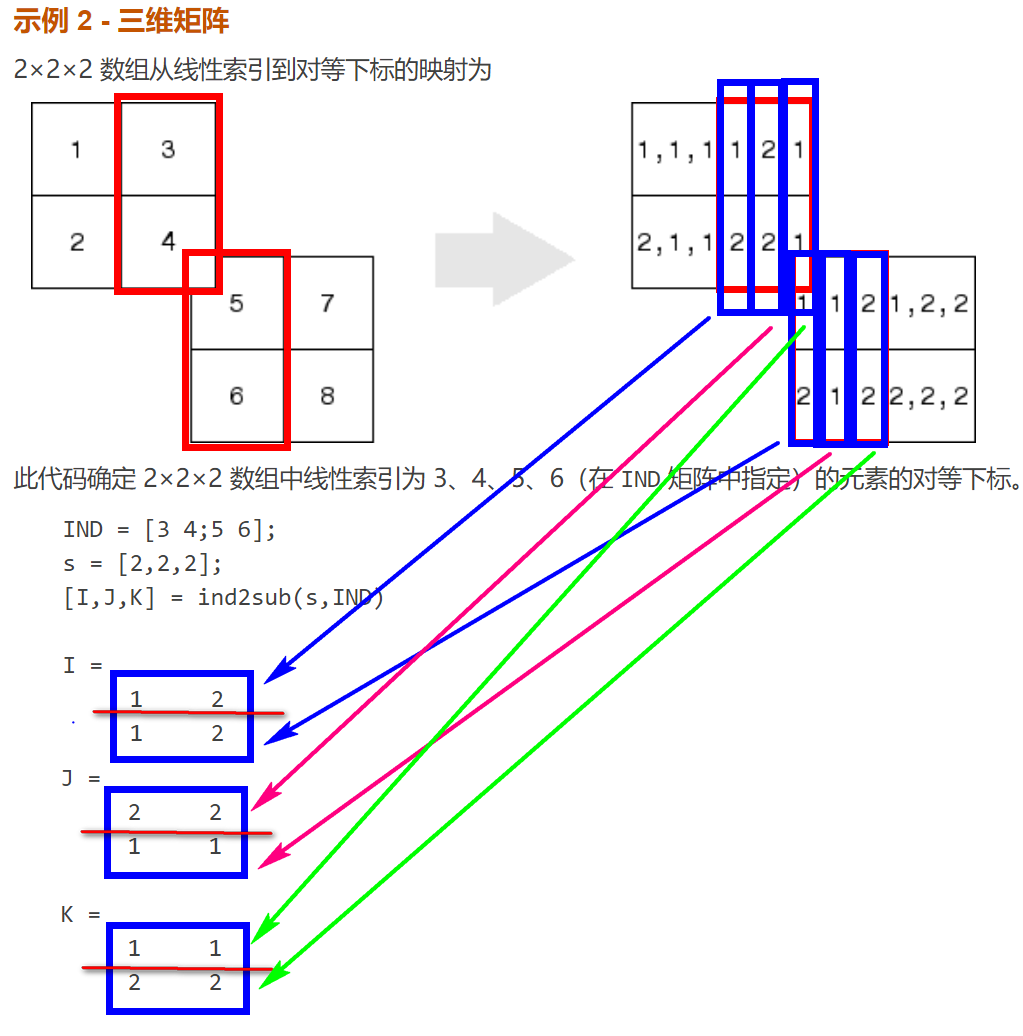降维输出
对 N 维矩阵调用 ind2sub 时，通常应在调用中提供 N 个输出参数：矩阵的每个维度一个参数。此示例显示在对某个三维矩阵调用 ind2sub 时返回三个、两个和一个输出时发生的情况。  该矩阵为 2×2×2 矩阵，并且线性索引从 1 到 8：
dims = [2 2 2];indices = [1 2 3 4 5 6 7 8];  具有三个输出的 ind2sub 调用返回 2×2×2 矩阵的预期下标： [rowsub colsub pagsub] = ind2sub(dims, indices) rowsub = 1 2 1 2 1 2 1 2 colsub = 1 1 2 2 1 1 2 2 pagsub = 1 1 1 1 2 2 2 2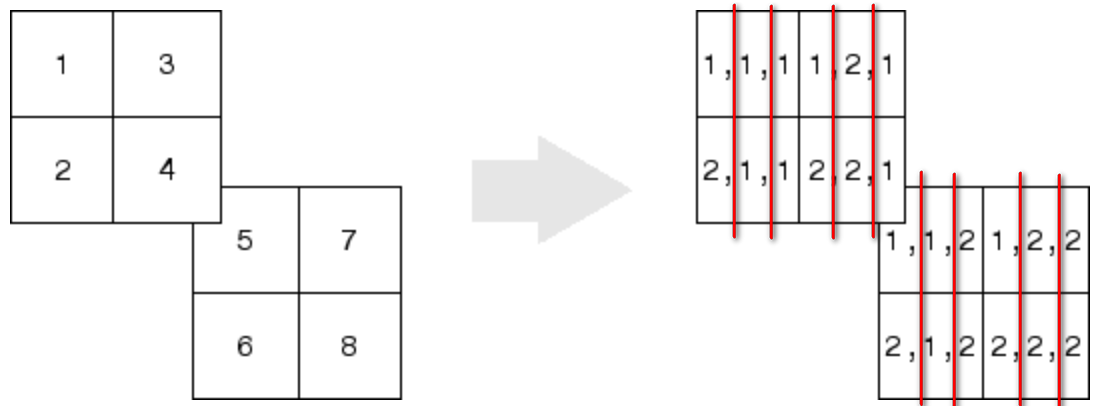如果您仅指定两个输出（行和列），ind2sub 仍将返回每个指定索引的下标，但会从矩阵中删除第三个维度，并改为返回一个二维的 2×4 矩阵的下标：
[rowsub colsub] = ind2sub(dims, indices) rowsub = 1 2 1 2 1 2 1 2 colsub = 1 1 2 2 3 3 4 4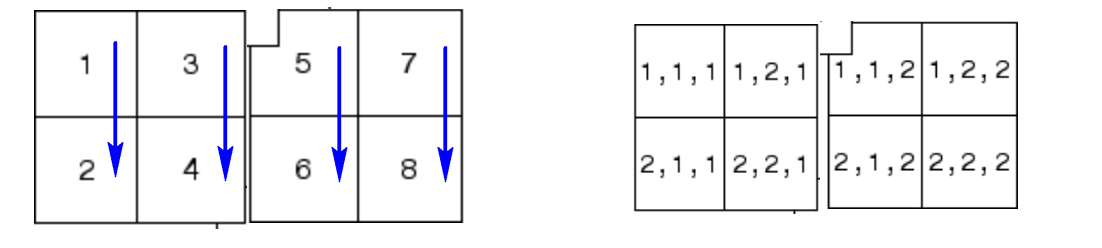如果您指定一个输出（行），ind2sub 将从矩阵中删除第二个维度和第三个维度，并改为返回一个一维的 1×8 矩阵的下标：
[rowsub] = ind2sub(dims, indices) rowsub = 1 2 3 4 5 6 7 8
展开全文squeeze
• 在matlab中，矩阵的存储是按列优先，sub2ind函数将矩阵中指定元素的行列下标转换成存储的序号，即线性索引号。下面，我们举例子进行说明。 1 建立一个3*4*2的矩阵 rng(0,'twister'); % Initialize random number ...matlab
• sub2ind函数是MATLAB中对矩阵索引号检索的函数，用法如下： A=rand(5) A = 0.6850 0.0867 0.2290 0.1006 0.4053 0.5979 0.7719 0.6419 0.2941 0.1048 0.7894 0.2057 0.4845 0.2374 0.1123 0.3677 0.3883 0.1518 ...matlab
• 矩阵的索引转换，sub2ind（）是双下标索引值转换为单下标索引值，ind2sub（）则相反。具体是什么意思呢。通过下面程序来看： 首先我们构造了一个矩阵，然后应用sub2ind（）函数进行转换，例子中是，第二行第三列...
• su2ind函数是对矩阵索引号检索的函数，ans=sub2ind(size,I,J) 其中第一个参数size表示矩阵的行数和列数，I是转换矩阵的行标，J是转换矩阵的列标。I和J的行列数必须相同。最终结果ans对应I,J对应行列在整个矩阵中的...
• ## sub2ind函数用法 MATLAB

千次阅读 多人点赞 2018-03-26 08:40:53
sub2ind 是下标与索引相互转换用的索引就是把整个向量或矩阵重拍成一维向量后所处的位置。如A=[1:3;4:6]; 排序后就是 1 4 2 5 3 6 其中1的索引就是1；4的索引就是2，以此类推。2.例子在命令窗口输入：&gt;...matlab
• 使用方法 编辑本段回目录sub2ind函数是把下标值转化相应的单索引值。 ind = sub2ind(siz,i,j) 返回大小为siz矩阵中等价于行和列下标i和j的线性索引值。siz是含有ndim(a)个元素的向量，其中siz(1)是行数，siz(2)是列...
• var sub2ind = require ( 'compute-sub2ind' ) ; sub2ind( x, i, j ) 将下标转换为线性索引。 var matrix = require ( 'dstructs-matrix' ) ; var mat = matrix ( [ 3 , 2 ] ) ; /* Matrix Subscripts Indices ...JavaScript
• MATLAB--矩阵的引用方式 矩阵元素的引用 sub2ind函数 ind2sub函数 利用冒号表达式获得子矩阵 end 运算符 利用空矩阵删除矩阵的元素 reshape函数 A( : ) 最后 矩阵元素的引用 通过下标来引用矩阵的元素。 A(3,2)表示A...matlab 机器学习 算法
• ## MATLAB sub2ind函数用法

万次阅读 多人点赞 2018-05-17 09:29:37
sub2ind函数是MATLAB中对矩阵索引号检索的函数，用法如下：&gt;&gt; A=rand(5)A = 0.6850 0.0867 0.2290 0.1006 0.4053 0.5979 0.7719 0.6419 0.2941 0.1048 0.7894 0.2057 0.4845 0.2374 0...
• 标识方法 在matlab软件中，矩阵的元素可以使用三种方式进行标识： 全下标标识法 最经典的标识方法，使用行下标与列下标的组合用以标识单个元素 单下标标识法 矩阵的后台存储形式是...sub2ind函数 A = [1 2 3; 4 5 6matlab
• ind = array2ind(SIZE, COORDINATES_AS_ARRAY) 例子： g = 魔法（5）； ind = array2ind([5 5], [3 2]) 克（指数） 返回与调用相同的值 g(3,2) 当矩阵元素的坐标存储为数组并避免当前方法时很有用： b = [3 2]...matlab
• sub2ind与ind2sub函数 A = [1 2 3; 4 5 6;7,8,9]; &gt;&gt; f=sub2ind(size(A), 2, 3) f =  8 即把矩阵A中第二行第三列的元素的全下标标识（2,3）转换为对应的单下标标识8，即该元素从第一列顺次数过去是第...
• 将下标转换为线性索引 语法 ...linearInd=sub2ind(matrixSize,rowSub,colSub...linearInd=sub2ind(matrixSize,rowSub,colSub)为大小是matrixSize的矩阵返回与行和列下标rowSub和colSub等效的线性索引。matrixSi...
• MATLAB中的索引和下标转换的两个函数，ind2subsub2ind。实际应用中用途很广，初学者学习分享matlab 索引
• sub2ind 将下标转换为线性索引。 一、语法 linearInd = sub2ind(matrixSize, rowSub, colSub) 说明 sub2ind 为大小是 matrixSize 的矩阵返回与行和列下标 rowSub 和 colSub 等效的线性索引。matrixSize 输入是一个...
• 目录 一.语法 1.输入参数 ...ind = sub2ind(sz,I1,I2,...,In) 1.输入参数 sz-数组的大小 正整数向量 数组的大小，指定为正整数向量。此向量的每个元素指示对应维度的大小。例如，[2 3 4]定义 2×...
• ## matlab ind2sub和sub2ind

千次阅读 2014-12-23 14:40:29
ind2subsub2ind是matlab中下标与索引相互转换用的两个函数。 ind代表索引（index）。sub代表下标（subscript），即行列值。 在命令窗口输入： >> A=[4 7 2 9 8;3 9 1 4 3;1 5 9 6 4;8 3 7 1 0] A = 4 7 2 ...matlab命令 matlab 索引
• matlab开发-Sub2Ind4UplineIndexFromSubscriptsOfUpperTriangularMetrixOnlyElementsAboveCollector。二维上矩阵给定下标的单个索引（对角线上无元素）
• ## sub2ind和ind2sub

千次阅读 2016-05-28 20:11:12
1、sub2ind A=[1 4 5 7；2 4 3 0；1 3 5 8] A= 1 4 5 7  2 4 3 0  1 3 5 8] b=sub2ind(size(A),2,2) b=5 c=sub2ind(size(A),3,3) c=9 sub2ind是找到矩阵A对应位置的索引号（将矩阵下标subscribe转换成索...matlab
• IND = SUB2IND4UP(I, J) 返回相当于行和列下标 I 和 J 的线性索引让 ind 是某个上三角矩阵的条目的索引向量。 垂直选择条目，以便： ind = 1与条目（1、2）相关联ind = 2 与条目 (1, 3) 相关联ind = 3 与条目 (2, 3...matlab
• sub2ind函数是MATLAB中对矩阵索引号检索的函数，用法如下： >> A=rand(5) A = 0.6850 0.0867 0.2290 0.1006 0.4053 0.5979 0.7719 0.6419 0.2941 0.1048 0.7894 0.2057 0.4845 0.237......